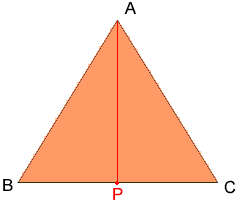My name is Rosa and I'm the eight grade. My question is If Each side of an equilateral triangle is 10 m. What is the height? Hi Rosa, I drew your triangle and labeled the vertices A, B and C so that I can describe what to do.Let P me the midpoint of the side BC, then the triangles ABP and APC are congruent. This tells you that the angles measures of the BPA and APC are equal. Since their sum is 180 degrees, each of them is 90 degrees. Thus triangle APC is a right triangle. The length of CA is 10 m, and the length of PC is 5 m, and hence you can use Pythagoras' theorem to find the length of AP, which is the height of the triangle ABC. Cheers, Penny Go to Math Central